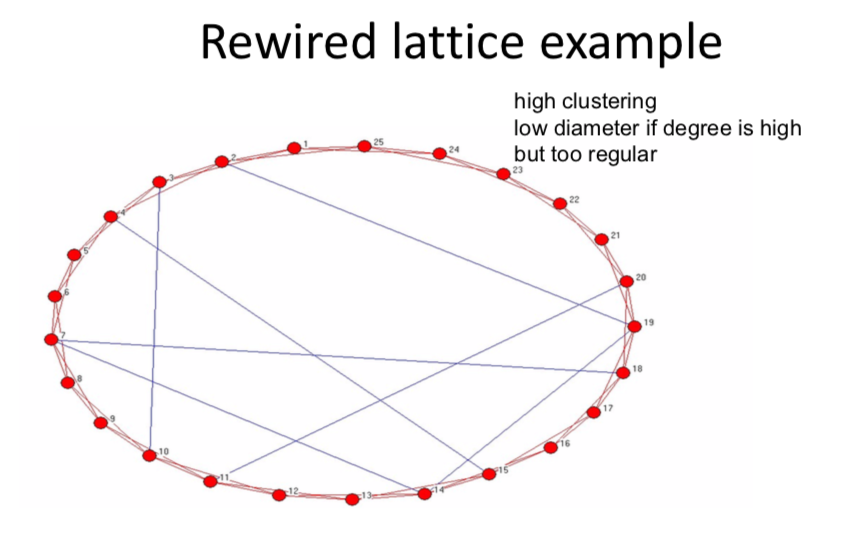# Outline¶

• Part 1: Background and Fundamentals

• Definitions and Characteristics of Networks(1,2)
• Empirical Background(3)
• Part 2: Network Formation

• Random Network Models (4,5)
• Strategic Network Models (6,11)
• Part 3: Networks and Behavior

• Diffusion and Learning (7,8)
• Games on Networks (9)

# Other Static Models¶

• Models to generate clustering

• Models to generate other than Poisson degree distributions

• Models to fit to data

# Rewired lattice ‐Watts and Strogatz 98¶

• Erdos‐Renyi model misses clustering
• clustering is on the order of p; going to 0 unless average degree is becoming infinite (and highly so...)
• Start with ring‐lattice and then randomly pick some links to rewire
• start with high clustering but high diameter – as rewire enough links, get low diameter
• don’t rewire too many, keep high clusteringSo here's just picture I drew of here of a set, a set of nodes. So 25 nodes in a ring lattice. And so initially they're connected, each node is connected to its two immediate neighbors. So if we look here we have node one, and it's connected two and three, and also connected to 25 and 24. Right? So it's got these connections going here and here. and then, each one of the nodes is, in terms of these in terms of the red, have connections to the, the different neighbors, okay? So we've, we've, we are connected to your 2 neighbors. What that does is, in terms of this original lattice is give you very high clustering. So 1 is connected to both 2 and 3, and 2 and 3 are connected to each other. 1 is connected to the 2 and 25, and 2 and 25 are connected to each other. And so forth. So the clustering is is high when you start. And then what you can do is is actually what I've done is this picture is rewire the links but just to had a few random links. Links. So let's just stick a few random links in a network. And so what happens is initially if you wanted to get without these initial, without these random links here, if you wanted to get from node one to node 15 your path length would be quite far. Right? You'd have to go sort of marching around the circle. Your path length, especially if you expanded this thing to be a much larger graph, your path link would be quite far.

By putting in these few connections, these extra ones, now to get from 1 to 15, you just go, you know, you've got a, a fairly short path, right? You're connected in a, at a distance 4. to get from one to 14, you know, you're connected at a distance three. So a few of these extra things allow you to get so one can get to ten now through, in, in just two hops, and so forth. So a few extra links actually dramatically shortens the average path length. but it doesn't change the clustering too much. Right? And if you just, you know, deleted some links, but some of these new ones in, as long as you're keeping it in the sort of sweet spot, what Watson Strogeth noticed Wwas that you could just do a little bit of rewiring that shrinks the diameter dramatically, and yet you keep reasonably high clustering. Okay? Now of course, if you look at this network, and you look at the, the shape of it, it's still not going to match a lot of real-world networks. why not? Well, because, you know, a lot of these nodes basically have degree four still, or, or, you know, fairly regular. So it's, it's not going to fat, match the fat tails and other kinds of things that we actually observe, but what it does do is it begins to sort of answer some of the questions so that if that for some reason we had high clustering on our, on, to start with in terms of some local level And then we just add a few random links on top of that. We can get at least two features in common, right, so it gives us some explanation of how these things can begin to arise in common. You don't need many random links to actually shorten average path length dramtically. Now, you know, this model is far from one we would want to take to data. But it begins to put some different things together. And what we'll going to begin to do now is start to enrich the models so that they're at the level where we can begin to look at different things, put them in, take them to data and then ask things about, you know, are we reproducing a lot of the features that we actually observe in, in real world networks. So, we'll, we'll take a more detailed look at random networks.